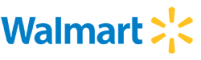New update is available. Click here to update.
Topics

# Pair Product Div by K

Moderate0/80
Average time to solve is 35m
0 upvote## Problem statement

Given a integer array nums of length ‘N’ and an integer ‘K’, return the number of pairs ‘(i, j)’ such that:

1 <= ‘i’ < ‘j’ <= ‘N’ and ‘nums[i] * nums[j]’ is divisible by ‘K’.

EXAMPLE :
``````‘N’ = 5, ‘K’ = 4, ‘nums’ = [2, 6, 1, 7, 8]
Output: 5
Explanation: (1, 2), (1, 5), (2, 5), (3, 5), and (4, 5) pairs have their products divisible by K.
``````
Detailed explanation ( Input/output format, Notes, Images )
Constraints :
``````1 <= 'T' <= 10
1 <= 'N' <= 10^5
1 <= 'K' <= 10^5
1 <= ‘nums[i]’ <= 10^6
It is guaranteed that sum of ‘N’ over all test cases is <= 10^5
Time Limit: 1 sec
``````
Sample Input 1 :
``````2
4 5
1 2 3 4
5 2
1 2 3 4 5
``````
Sample Output 1 :
``````0
7
``````
Explanation Of Sample Input 1 :
``````For the first test case, there is no pair of indices whose corresponding product is divisible by 5.

For the second test case, the seven pairs of indices whose corresponding products are divisible by two are
(1, 2), (1, 4), (2, 3), (2, 4), (2, 5), (3, 4), and (4, 5).
Their products are 2, 4, 6, 8, 10, 12, and 20 respectively.
Other pairs such as (1, 3) and (3, 5) have products 3 and 15, respectively, which are not divisible by 2.
``````
Sample Input 2 :
``````2
7 3
3 2 6 1 8 4 1
9 6
8 10 2 5 9 6 3 8 2
``````
Sample Output 2 :
``````11
18
``````Console